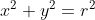## General Forms

What shape does the shown equation represent?Hint
The standard form of a sphere equation is:
$$(x-h)^2+(y-k)^2+(z-m)^2=r^2$$$where $$r$$ is the sphere’s radius, and $$h,k,\&\: m$$ are the respective $$x,y,z$$ coordinates that define the sphere’s center point. Hint 2 The standard form of a circle equation is: $$(x-h)^2+(y-k)^2=r^2$$$
where $$r$$ is the circle’s radius, and $$h\:\&\:k$$ are the respective $$x\:\&\:y$$ coordinates that define the circle’s center point.
The standard form of a sphere equation is:
$$(x-h)^2+(y-k)^2+(z-m)^2=r^2$$$where $$r$$ is the sphere’s radius, and $$h,k,\&\: m$$ are the respective $$x,y,z$$ coordinates that define the sphere’s center point. The standard form of a circle equation is: $$(x-h)^2+(y-k)^2=r^2$$$
where $$r$$ is the circle’s radius, and $$h\:\&\:k$$ are the respective $$x\:\&\:y$$ coordinates that define the circle’s center point.

If a circle were centered at the origin $$(h=0\:\&\:k=0)$$ , then the general form is:
$$x^2+y^2=r^2$$\$
Circle